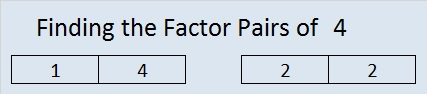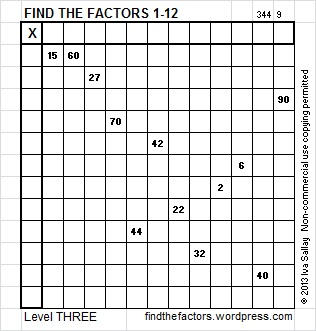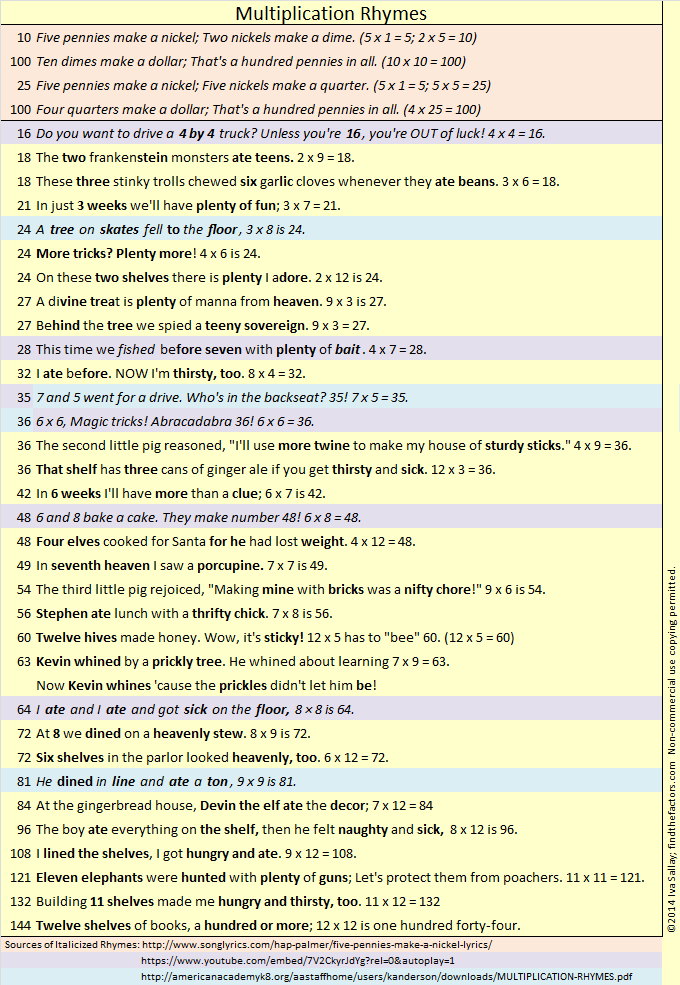# 138 and Divisibility Tricks 4 You

138 is a composite number. Factor pairs: 138 = 1 x 138, 2 x 69, 3 x 46, or 6 x 23. Factors of 138: 1, 2, 3, 6, 23, 46, 69, 138. Prime factorization: 138 = 2 x 3 x 23.138 is never a clue in the FIND THE FACTORS puzzles.

———————————————————————————

After you learned some basic division facts, you probably realized:

• 2 will divide evenly into any EVEN whole number.
• 5 will divide evenly into whole numbers ending in 0 or 5.
• 10 will divide evenly into whole numbers ending in 0.

These three rules are related to each other. All of them are true because we use base ten in our numbering system, and the prime factorization of 10 is 2 x 5.

If you needed to find the factors of a 33-digit whole number, you would be able to tell if 2, 5, or 10 divide evenly into it  just by looking at the last digit. 33-digits is more than a standard calculator can handle, but no matter how many digits a whole number has, as long as you can see the very last one, you can apply those three simple divisibility rules to know if 2, 5, or 10 are factors. Thus you will be able to do something a calculator can’t.

But wait, there are even more divisibility tricks if you can see the last TWO digits of the whole number!• 10 squared, better known as 100, divides evenly into any whole number ending in 00.
• 5 x 5 = 25 which divides evenly into any whole number ending in 00, 25, 50, or 75.
• 2^2 (AKA 4) divides evenly into a whole number if the final two digits can be divided evenly by 4.

How can one tell if the last two digits of a whole number are divisible by 4 (without actually dividing by 4)? I’ll show you how: I’ve put the 25 possible 2-digit multiples of 4 into one of two lists:

• 00, 04, 08, 20, 24, 28, 40, 44, 48, 60, 64, 68, 80, 84, 88
• 12, 16, 32, 36, 52, 56, 72, 76, 92, 96

Notice in the first list ALL the digits are even and the last digit (0, 4, or 8) can be divided evenly by 4.

Then look at the second list. The first digit is always odd and the last digit is either 2 or 6 (the only two even digits that are not divisible by 4).

Hmm. I think we can rewrite the divisibility rule for 4:

• 4 (AKA 2^2) divides evenly into a whole number if the last two digits are even and the final digit is divisible by 4 (the last digit is 0, 4, or 8).
• 4 divides evenly into any whole number whose next to the last digit is odd if the final digit is even but not divisible by 4 (the last digit is 2 or 6).

The rewritten divisibility rule is longer to read but takes a little less time to implement so you will have to decide which version of the rule works best for you. Either trick takes much less time than dividing some really long whole number by 4 or dividing by 2 twice.

Now I’m on to thinking about what the last THREE digits tell us.

# 4 Rhyme and Rhythm

4  is the first composite number, and it is 2 squared. 4 = 1 x 4 or 2 x 2. Factors of 4: 1, 2, 4. Prime factorization: 4 = 2 x 2, which can also be written 4 = 2².

Since √4 = 2, a whole number, 4 is a perfect square.When 4 is a clue in the FIND THE FACTORS puzzles, the factors might be 1 and 4 or they might be 2 and 2.

—————————————————————–

Seventeen years ago, when my daughter was learning the multiplication facts, I came across a rhyme that taught one fact:

5, 6, 7, 8……….56 is 7 times 8.

Coincidentally,

1, 2, 3, 4……….I know twelve is 3 times 4.

3 x 4 = 12 isn’t as difficult to remember as 7 x 8 = 56. Still I find it fun to notice the relationship between counting to eight and these two multiplication facts. I enjoyed the rhymes mentioned in my 10/31/2013 post, and I have found yet another site with rhymes for learning the multiplication facts. Two rhymes similar to these 12 and 56 counting rhymes were even included!  The site is : http://www.teacherweb.com/NY/Quogue/MrsLevy/MULTIPLICATION-RHYMES.pdf

Some children have no problem memorizing number facts, but for some children, a rhyme makes learning the facts more fun and much easier.  Even though I already know all of the basic multiplication facts, I am going to memorize these rhymes simply because I personally enjoy them.  I also know I will have at least one opportunity every week to share them with someone trying to memorize the facts: Already when a student asks me, “what’s 7 x 8?” I always answer in rhyme.

Besides the two rhymes listed above, my favorites are:

 Times One: Mirror, mirror look and see, it’s the other number, not me. Times Zero:  Zero is always the hero Six times six / Magic tricks / Abracadabra / thirty-six A tree on skates fell on the floor / Three times eight is twenty-four. A 4 by 4 is a big machine, Iʼm going to get one when Iʼm 16.

This week I even wrote one myself:  Twelve times twelve / Is a dozen dozen / A gross one forty-four / Just ask my cousin.

When a very young child loves a rhyme, he or she will want to hear it over and over again. Mother Goose rhymes have been enriching lives for years. Being able to fully comprehend what the rhyme is about isn’t necessary at first so even preschoolers can be introduced to these rhymes.

Robin Liner writes a blog (crazygoodreaders.wordpress.com) that discusses reading and dyslexia.  On October 5, 2013 , she wrote Rhythm and Rhyme: A Phonological Power Tool.  She wrote, “Rhymes provide subconscious clues.” That means someone is more likely to get an answer right when that answer rhymes with the question. What a fun and powerful way to learn!

Much of what she wrote not only applies to learning to read but also to learning math, science, history, ……. anything.

Twice a week I put 6 new puzzles in an excel file that is attached to this blog. The puzzles can be solved using logic and knowledge of the multiplication table. Here is one of the puzzles I created this week.Click on this link, 12 Factors 2013-11-07,  for more puzzles and the previous week’s solutions. How do you solve the puzzles? Place the numbers 1 – 12 in both the top row and the first column so that those numbers are the factors of the given clues.

Update: I like the idea of using rhymes to learn multiplication facts so much that I compiled my own list: